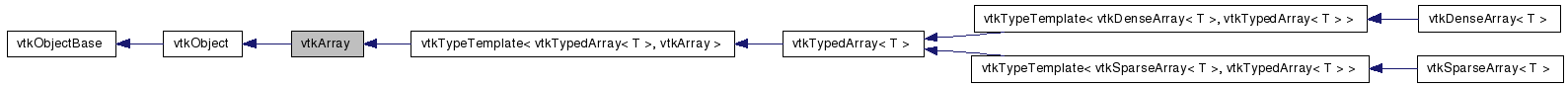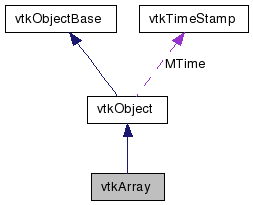vtkArray Class Reference

#include <vtkArray.h>

Inheritance diagram for vtkArray:[legend]
Collaboration diagram for vtkArray:[legend]

Detailed Description

Abstract interface for N-dimensional arrays.

vtkArray is the root of a hierarchy of arrays that can be used to store data with any number of dimensions. It provides an abstract interface for retrieving and setting array attributes that are independent of the type of values stored in the array - such as the number of dimensions, extents along each dimension, and number of values stored in the array.

To get and set array values, the vtkTypedArray template class derives from vtkArray and provides type-specific methods for retrieval and update.

Two concrete derivatives of vtkTypedArray are provided at the moment: vtkDenseArray and vtkSparseArray, which provide dense and sparse storage for arbitrary-dimension data, respectively. Toolkit users can create their own concrete derivatives that implement alternative storage strategies, such as compressed-sparse-row, etc. You could also create an array that provided read-only access to 'virtual' data, such as an array that returned a Fibonacci sequence, etc.

vtkTypedArray, vtkDenseArray, vtkSparseArray
Thanks:
Tests:
vtkArray (Tests)

Definition at line 61 of file vtkArray.h.

Public Types

enum  { DENSE = 0, SPARSE = 1 }
typedef vtkObject Superclass

Public Member Functions

virtual const char * GetClassName ()
virtual int IsA (const char *type)
void PrintSelf (ostream &os, vtkIndent indent)
virtual vtkArrayExtents GetExtents ()=0
vtkIdType GetDimensions ()
vtkIdType GetSize ()
virtual vtkIdType GetNonNullSize ()=0
void SetDimensionLabel (vtkIdType i, const vtkStdString &label)
vtkStdString GetDimensionLabel (vtkIdType i)
virtual void GetCoordinatesN (const vtkIdType n, vtkArrayCoordinates &coordinates)=0
virtual vtkVariant GetVariantValueN (const vtkIdType n)=0
virtual void SetVariantValueN (const vtkIdType n, const vtkVariant &value)=0
virtual vtkArrayDeepCopy ()=0
void Resize (vtkIdType i)
void Resize (vtkIdType i, vtkIdType j)
void Resize (vtkIdType i, vtkIdType j, vtkIdType k)
void Resize (const vtkArrayExtents &extents)
vtkVariant GetVariantValue (vtkIdType i)
vtkVariant GetVariantValue (vtkIdType i, vtkIdType j)
vtkVariant GetVariantValue (vtkIdType i, vtkIdType j, vtkIdType k)
virtual vtkVariant GetVariantValue (const vtkArrayCoordinates &coordinates)=0
void SetVariantValue (vtkIdType i, const vtkVariant &value)
void SetVariantValue (vtkIdType i, vtkIdType j, const vtkVariant &value)
void SetVariantValue (vtkIdType i, vtkIdType j, vtkIdType k, const vtkVariant &value)
virtual void SetVariantValue (const vtkArrayCoordinates &coordinates, const vtkVariant &value)=0
virtual void CopyValue (vtkArray *source, const vtkArrayCoordinates &source_coordinates, const vtkArrayCoordinates &target_coordinates)=0
virtual void CopyValue (vtkArray *source, const vtkIdType source_index, const vtkArrayCoordinates &target_coordinates)=0
virtual void CopyValue (vtkArray *source, const vtkArrayCoordinates &source_coordinates, const vtkIdType target_index)=0

Static Public Member Functions

static int IsTypeOf (const char *type)
static vtkArraySafeDownCast (vtkObject *o)
static vtkArrayCreateArray (int StorageType, int ValueType)

vtkArray ()
~vtkArray ()

Member Typedef Documentation

 typedef vtkObject vtkArray::Superclass

Reimplemented from vtkObject.

Definition at line 64 of file vtkArray.h.

Member Enumeration Documentation

 anonymous enum

Enumerator:
 DENSE Used with CreateArray() to create dense arrays. SPARSE Used with CreateArray() to create sparse arrays.

Definition at line 68 of file vtkArray.h.

Constructor & Destructor Documentation

 vtkArray::vtkArray ( ) [protected]

 vtkArray::~vtkArray ( ) [protected]

Member Function Documentation

 virtual const char* vtkArray::GetClassName ( ) [virtual]

Reimplemented from vtkObject.

 static int vtkArray::IsTypeOf ( const char * name ) [static]

Return 1 if this class type is the same type of (or a subclass of) the named class. Returns 0 otherwise. This method works in combination with vtkTypeRevisionMacro found in vtkSetGet.h.

Reimplemented from vtkObject.

 virtual int vtkArray::IsA ( const char * name ) [virtual]

Return 1 if this class is the same type of (or a subclass of) the named class. Returns 0 otherwise. This method works in combination with vtkTypeRevisionMacro found in vtkSetGet.h.

Reimplemented from vtkObject.

 static vtkArray* vtkArray::SafeDownCast ( vtkObject * o ) [static]

Reimplemented from vtkObject.

 void vtkArray::PrintSelf ( ostream & os, vtkIndent indent ) [virtual]

Methods invoked by print to print information about the object including superclasses. Typically not called by the user (use Print() instead) but used in the hierarchical print process to combine the output of several classes.

Reimplemented from vtkObject.

Reimplemented in vtkDenseArray< T >, vtkSparseArray< T >, and vtkTypedArray< T >.

 static vtkArray* vtkArray::CreateArray ( int StorageType, int ValueType ) [static]

Creates a new array where StorageType is one of vtkArray::DENSE or vtkArray::SPARSE, and ValueType is one of VTK_CHAR, VTK_UNSIGNED_CHAR, VTK_SHORT, VTK_UNSIGNED_SHORT, VTK_INT, VTK_UNSIGNED_INT, VTK_LONG, VTK_UNSIGNED_LONG, VTK_DOUBLE, VTK_ID_TYPE, or VTK_STRING. The caller is responsible for the lifetime of the returned object.

 void vtkArray::Resize ( vtkIdType i )

Resizes the array to the given extents (number of dimensions and size of each dimension). Note that concrete implementations of vtkArray may place constraints on the the extents that they will store, so you cannot assume that GetExtents() will always return the same value passed to Resize(). The contents of the array are undefined after calling Resize() - you should initialize its contents accordingly. In particular, dimension-labels will be undefined, dense array values will be undefined, and sparse arrays will be empty.

 void vtkArray::Resize ( vtkIdType i, vtkIdType j )

Resizes the array to the given extents (number of dimensions and size of each dimension). Note that concrete implementations of vtkArray may place constraints on the the extents that they will store, so you cannot assume that GetExtents() will always return the same value passed to Resize(). The contents of the array are undefined after calling Resize() - you should initialize its contents accordingly. In particular, dimension-labels will be undefined, dense array values will be undefined, and sparse arrays will be empty.

 void vtkArray::Resize ( vtkIdType i, vtkIdType j, vtkIdType k )

Resizes the array to the given extents (number of dimensions and size of each dimension). Note that concrete implementations of vtkArray may place constraints on the the extents that they will store, so you cannot assume that GetExtents() will always return the same value passed to Resize(). The contents of the array are undefined after calling Resize() - you should initialize its contents accordingly. In particular, dimension-labels will be undefined, dense array values will be undefined, and sparse arrays will be empty.

 void vtkArray::Resize ( const vtkArrayExtents & extents )

Resizes the array to the given extents (number of dimensions and size of each dimension). Note that concrete implementations of vtkArray may place constraints on the the extents that they will store, so you cannot assume that GetExtents() will always return the same value passed to Resize(). The contents of the array are undefined after calling Resize() - you should initialize its contents accordingly. In particular, dimension-labels will be undefined, dense array values will be undefined, and sparse arrays will be empty.

 virtual vtkArrayExtents vtkArray::GetExtents ( ) [pure virtual]

Returns the extents (the number of dimensions and size along each dimension) of the array.

Implemented in vtkDenseArray< T >, and vtkSparseArray< T >.

 vtkIdType vtkArray::GetDimensions ( )

Returns the number of dimensions stored in the array. Note that this is the same as calling GetExtents().GetDimensions().

 vtkIdType vtkArray::GetSize ( )

Returns the number of values stored in the array. Note that this is the same as calling GetExtents().GetSize(), and represents the maximum number of values that could ever be stored using the current extents. This is equal to the number of values stored in a dense array, but may be larger than the number of values stored in a sparse array.

 virtual vtkIdType vtkArray::GetNonNullSize ( ) [pure virtual]

Returns the number of non-null values stored in the array. Note that this value will equal GetSize() for dense arrays, and will be less-than-or-equal to GetSize() for sparse arrays.

Implemented in vtkDenseArray< T >, and vtkSparseArray< T >.

 void vtkArray::SetDimensionLabel ( vtkIdType i, const vtkStdString & label )

Sets the label for the i-th array dimension.

 vtkStdString vtkArray::GetDimensionLabel ( vtkIdType i )

Returns the label for the i-th array dimension.

 virtual void vtkArray::GetCoordinatesN ( const vtkIdType n, vtkArrayCoordinates & coordinates ) [pure virtual]

Returns the coordinates of the n-th value in the array, where n is in the range [0, GetNonNullSize()). Note that the order in which coordinates are visited is undefined, but is guaranteed to match the order in which values are visited using vtkTypedArray::GetValueN() and vtkTypedArray::SetValueN().

Implemented in vtkDenseArray< T >, and vtkSparseArray< T >.

 vtkVariant vtkArray::GetVariantValue ( vtkIdType i ) [inline]

Returns the value stored in the array at the given coordinates. Note that the number of dimensions in the supplied coordinates must match the number of dimensions in the array.

Definition at line 202 of file vtkArray.h.

 vtkVariant vtkArray::GetVariantValue ( vtkIdType i, vtkIdType j ) [inline]

Returns the value stored in the array at the given coordinates. Note that the number of dimensions in the supplied coordinates must match the number of dimensions in the array.

Definition at line 207 of file vtkArray.h.

 vtkVariant vtkArray::GetVariantValue ( vtkIdType i, vtkIdType j, vtkIdType k ) [inline]

Returns the value stored in the array at the given coordinates. Note that the number of dimensions in the supplied coordinates must match the number of dimensions in the array.

Definition at line 212 of file vtkArray.h.

 virtual vtkVariant vtkArray::GetVariantValue ( const vtkArrayCoordinates & coordinates ) [pure virtual]

Returns the value stored in the array at the given coordinates. Note that the number of dimensions in the supplied coordinates must match the number of dimensions in the array.

Implemented in vtkTypedArray< T >.

 virtual vtkVariant vtkArray::GetVariantValueN ( const vtkIdType n ) [pure virtual]

Returns the n-th value stored in the array, where n is in the range [0, GetNonNullSize()). This is useful for efficiently visiting every value in the array. Note that the order in which values are visited is undefined, but is guaranteed to match the order used by vtkArray::GetCoordinatesN().

Implemented in vtkTypedArray< T >.

 void vtkArray::SetVariantValue ( vtkIdType i, const vtkVariant & value ) [inline]

Overwrites the value stored in the array at the given coordinates. Note that the number of dimensions in the supplied coordinates must match the number of dimensions in the array.

Definition at line 217 of file vtkArray.h.

 void vtkArray::SetVariantValue ( vtkIdType i, vtkIdType j, const vtkVariant & value ) [inline]

Overwrites the value stored in the array at the given coordinates. Note that the number of dimensions in the supplied coordinates must match the number of dimensions in the array.

Definition at line 222 of file vtkArray.h.

 void vtkArray::SetVariantValue ( vtkIdType i, vtkIdType j, vtkIdType k, const vtkVariant & value ) [inline]

Overwrites the value stored in the array at the given coordinates. Note that the number of dimensions in the supplied coordinates must match the number of dimensions in the array.

Definition at line 227 of file vtkArray.h.

 virtual void vtkArray::SetVariantValue ( const vtkArrayCoordinates & coordinates, const vtkVariant & value ) [pure virtual]

Overwrites the value stored in the array at the given coordinates. Note that the number of dimensions in the supplied coordinates must match the number of dimensions in the array.

Implemented in vtkTypedArray< T >.

 virtual void vtkArray::SetVariantValueN ( const vtkIdType n, const vtkVariant & value ) [pure virtual]

Overwrites the n-th value stored in the array, where n is in the range [0, GetNonNullSize()). This is useful for efficiently visiting every value in the array. Note that the order in which values are visited is undefined, but is guaranteed to match the order used by vtkArray::GetCoordinatesN().

Implemented in vtkTypedArray< T >.

 virtual void vtkArray::CopyValue ( vtkArray * source, const vtkArrayCoordinates & source_coordinates, const vtkArrayCoordinates & target_coordinates ) [pure virtual]

Overwrites a value with a value retrieved from another array. Both arrays must store the same data types.

Implemented in vtkTypedArray< T >.

 virtual void vtkArray::CopyValue ( vtkArray * source, const vtkIdType source_index, const vtkArrayCoordinates & target_coordinates ) [pure virtual]

Overwrites a value with a value retrieved from another array. Both arrays must store the same data types.

Implemented in vtkTypedArray< T >.

 virtual void vtkArray::CopyValue ( vtkArray * source, const vtkArrayCoordinates & source_coordinates, const vtkIdType target_index ) [pure virtual]

Overwrites a value with a value retrieved from another array. Both arrays must store the same data types.

Implemented in vtkTypedArray< T >.

 virtual vtkArray* vtkArray::DeepCopy ( ) [pure virtual]

Returns a new array that is a deep copy of this array.

Implemented in vtkDenseArray< T >, and vtkSparseArray< T >.

The documentation for this class was generated from the following file:

Generated on Wed Jun 3 18:53:31 2009 for VTK by1.5.6